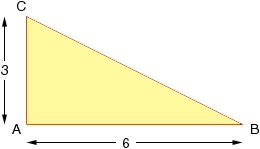Name: carissa Who is asking: Student Level: Secondary Question: How do you : Triangle ABC is right-angled at A. Calculate the length of the third side if b = 3 and c = 6, express the answer as a surd in its simplest form. Hi Carissa, Draw a diagram.The Theorem of Pythagoras says that, in a right angled triangle, the square of the hypotenuse is the sum of the squares of the other two sides. I this triangle the side BC is the hypotenuse and thus |BC|2 = 32 + 62 = 9 + 36 = 45 Hence |BC| = Sqrt(45) Express Sqrt(45) in its simplist form. Cheers, Harley Go to Math Central